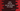# Java tutorial : Find all strong numbers in a range## Java program to check if a number is strong or not :

A number is called a strong number if the sum of factorials of each digit is equal to the number. In this tutorial, we will write one java program to find out all strong numbers from 1 to 100000. You can modify this program to get an input from the user and test if it is strong or not.

1. Using a for loop, we will check from 1 to 100000 if it is a strong number.
2. Method isItStrong(int) is using here to find out if the number is strong or not
3. Method getFactorial(int) is using here to find out the factorial of a number.
4. First of all, we are getting the rightmost digit of the number using ’%’ operator and calculating the factorial.
5. Sum of factorials is stored in a variable sum
6. On each iteration, right most digit of the number is removed : dividing it by 10
7. Finally, after the while loop ends, the total sum is checked with the number. If sum is equal to the number, it is a strong number .
8. If the number is detected as strong, return true. false otherwise.
9. If a number is strong, store it in a list.
10. After the check is done for all numbers, print out the list.

### Program :

``````import java.util.ArrayList;

public class Main {

public static void main(String[] args) {
ArrayList list = new ArrayList<>();

for (int i = 1; i <= 100000; i++) { if (isItStrong(i)) { //if the number is strong, store it in the ArrayList list.add(i); } } System.out.print(list); } /** * check if the number is strong or not * * @param userInputNo : input number * @return : true if userInputNo is strong */ static boolean isItStrong(int userInputNo) { int no = userInputNo; int sum = 0; while (no > 0) {
int digit = no % 10;
sum += getFactorial(digit);

no = no / 10;
}

if (sum == userInputNo)
return true;

return false;
}

/**
* get factorial of a number
*
* @param digit : number to find factorial
* @return : factorial of digit
*/
static int getFactorial(int digit) {
int fact = 1;
for (int j = digit; j > 1; j--) {
fact *= j;
}
return fact;
}
}``````

That’s all about strong number and how to find it using Java. Please drop us a comment below if you have any question on this tutorial.